# The SciPy Library¶

• The SciPy Library includes modules for
• optimization,
• interpolation,
• signal processing,
• linear algebra,
• sparse matrix representation,
• KD-Trees, Delaunay triangulation, convex hull computation
• statistics, and others

The SciPy library includes modules for optimization, interpolation, signal processing, linear algebra, statistics, sparse matrix representation and operation, among others. It also includes the spatial module, containing an implementations for the KD-Tree data structure (used on nearest-neighbor queries), Delaunay triangulation and convex hull computation.

## Optimization¶

• The optimize module presents algorithms for
• function minimization
• Broyden-Fletcher-Goldfarb-Shanno (BFGS)
• simulated annealing
• least-squares
• root finding
• fitting
In :
def f(x):
return x**2 + 10 * sin(x)

x = arange(-10, 10, 0.1)
plot(x, f(x), 'r-')

Out:
[<matplotlib.lines.Line2D at 0x4c3a150>]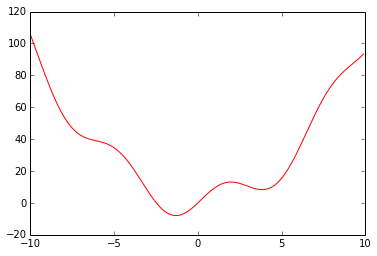### BFGS¶

In :
from scipy.optimize import fmin_bfgs

xmin = fmin_bfgs(f, 0)
xmin

Optimization terminated successfully.
Current function value: -7.945823
Iterations: 5
Function evaluations: 24

Out:
array([-1.30644003])
In :
plot(x, f(x), 'r-')
scatter([xmin],[f(xmin)], 10, color='red')
annotate('%f' % xmin,
xy=(xmin, f(xmin)), fontsize=12, xycoords='data',
xytext=(-90, -50), textcoords='offset points',
title('BFGS')

Out:
<matplotlib.text.Text at 0x4c18c10>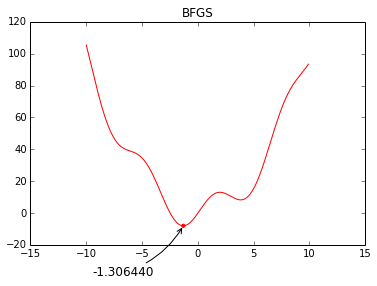#### Problem¶

Initialization and local minimima

In :
xmin = fmin_bfgs(f, 3, disp=0)
xmin

Out:
array([ 3.83746663])
In :
plot(x, f(x), 'r-')
scatter([xmin],[f(xmin)], 10, color='red')
annotate('%f' % xmin,
xy=(xmin, f(xmin)), fontsize=12, xycoords='data',
xytext=(40, -75), textcoords='offset points',
title('BFGS - local minima')

Out:
<matplotlib.text.Text at 0x4bb4510>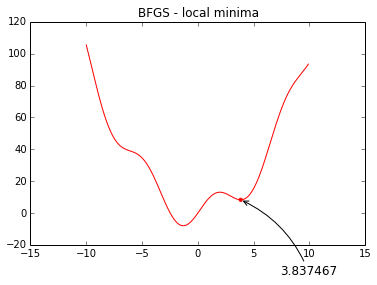#### Brute force¶

• Compute $f(x)$ for all $x$ in a grid
In :
from scipy.optimize import brute
grid = (-10, 10, 0.1)
xmin = brute(f, (grid,))
xmin

Out:
array([-1.30641113])
In :
plot(x, f(x), 'r-')
scatter([xmin],[f(xmin)], 10, color='red')
annotate('%f' % xmin,
xy=(xmin, f(xmin)), fontsize=12, xycoords='data',
xytext=(-90, -50), textcoords='offset points',
title('Brute force')

Out:
<matplotlib.text.Text at 0x5162c50>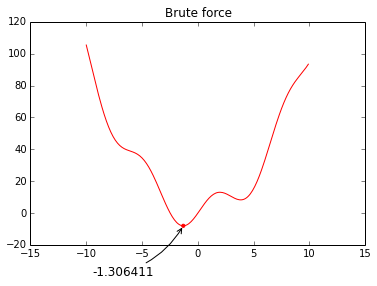#### Simulated Annealing¶

In :
from scipy.optimize import anneal

xmin, status = anneal(f, 3)
xmin, status

Warning: Cooled to 8.328202 at 3.78222236155 but this is not the smallest point found.

Out:
(-1.2167727301519746, 5)
In :
plot(x, f(x), 'r-')
scatter([xmin],[f(xmin)], 10, color='red')
annotate('%f' % xmin,
xy=(xmin, f(xmin)), fontsize=12, xycoords='data',
xytext=(-90, -50), textcoords='offset points',
title('Simulate Annealing')

Out:
<matplotlib.text.Text at 0x5193890>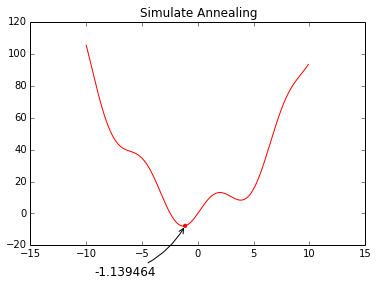## Linear algebra using scipy.linalg¶

• Linear algebra is particularly important to projective geometry
• Consider a 3D point $\mathbf{X}_i$
• Let $\mathrm{\tt P}$ be a camera matrix
• The projection of $\mathbf{X}_i$ on the camera plane is $\mathbf{x}_i = \mathrm{\tt P} \mathbf{X}_i$

Linear algebra is an essential mathematical tool for computer vision and machine learning. It is particularly important in problems involving projective geometry as in multiple view computer vision. Consider a 3D point $\mathbf{X}_i$, represented as a homogeneous 4-d array, and a projective matrix $\mathrm{\tt P}$, represented as a $3 \times 4$ array and corresponding to a camera. The projection of $\mathbf{X}_i$ on the camera’s image plane, $\mathbf{x}_i$, can be elegantly coded as:

In :
P = array([[  1.41598501e+03,  -3.53656018e+01,   6.29880366e+02,  -9.80199681e+02],
[ -1.66130187e+01,   1.52666051e+03,   2.41551888e+02,   2.34435820e+02],
[ -2.20274424e-01,   4.97475710e-03,   9.75425256e-01,   6.34871255e-01]])

X = array([-0.11652141, -0.24440939,  3.23347243,  1.])

x = dot(P, X)
x

Out:
array([ 900.15223192,  644.29279864,    3.81333274])

The dot function, for 2-D arrays, computes the matrix multiplication, and for 1-D arrays it calculates the inner product of vectors.

The pixel inhomogeneous coordinates can be recovered using:

In :
x_coord = x/x
y_coord = x/x
x_coord, y_coord

Out:
(236.05394389057571, 168.95792816629523)

### Singular value decompostion¶

• Several problems in multiple view geometry involve over-determined systems of equations
• Homography estimation
• 3D points triangulation
• $A\mathbf{x}$ is a homogeneous systems of linear equations
• Can be solved using Singular Value Decomposition (SVD)

Estimation problems in projective geometry involve the solution of over-determined systems of equations. More precisely, these problems are formalized as linear least-squares resolutions of homogeneous systems of linear equations in the form $A\mathbf{x}$, minimizing $A\mathbf{x}$ subject to $\| \mathbf{x} \| = 1$.

The minimization problem can be solved using singular value decomposition (SVD), a matrix decomposition particularly useful in numerical computations. SVD decomposes $A$ as $A = UDV^\intercal$ and the $\mathbf{x}$ solution corresponds to the last columns of $V$.

In :
def dlt_triangulation(ui, Pi, uj, Pj):
"""Hartley & Zisserman, 12.2"""
ui /= ui
xi, yi = ui, ui

uj /= uj
xj, yj = uj, uj

a0 = xi * Pi[2,:] - Pi[0,:]
a1 = yi * Pi[2,:] - Pi[1,:]
a2 = xj * Pj[2,:] - Pj[0,:]
a3 = yj * Pj[2,:] - Pj[1,:]

A = vstack((a0, a1, a2, a3))
U, s, VT = linalg.svd(A)
V = VT.T

X3d = V[:,-1]

return X3d/X3d


Examples of spatial.KDTree and linalg.svd will be presented in the Structure from Motion example.